Courses

# Short Answer Type Questions- Statistics Class 10 Notes | EduRev

## Class 10 : Short Answer Type Questions- Statistics Class 10 Notes | EduRev

The document Short Answer Type Questions- Statistics Class 10 Notes | EduRev is a part of the Class 10 Course Class 10 Mathematics by VP Classes.
All you need of Class 10 at this link: Class 10

Q1. Find the mode of the data:

 Marks 0-10 10-20 20-30 30-40 40-50 No. of students 3 12 32 20 6

Sol. Here, modal class is 20–30

f1 = 32, f2 = 20 and f0 = 12

Since, the lower limit of the modal class

l = 20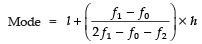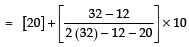[∵ h = 10]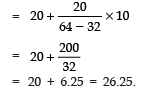Q2. The percentage marks obtained by 100 students in an examination are given below:

 Marks 30-35 35-40 40-45 45-50 50-55 55-60 60-65 Frequency 10 16 18 23 18 8 7

Find the median from the above data.

Sol. We have:

 Marks Frequency cf 30-35 10 10 + 0 = 10 35-40 16 16 + 10 = 26 40-45 18 18 + 26 = 44 45-50 23 23 + 44 = 67 50-55 18 18 + 67 = 85 55-60 8 8 + 85 = 93 60-65 7 7 + 93 = 100

Here,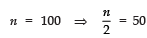∴ The median class is 45−50, such that

l = 45, cf = 44, f = 23 and h = 5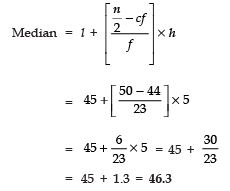Q3. Write a frequency distribution table for the following data:

 Marks Above 0 Above 10 Above 20 Above 30 Above 40 Above 50 No. of students 30 28 21 15 10 0

Sol. Since,

30 − 28 = 2
28 − 21 = 7
21 − 15 = 6
15 − 10 = 5
10 − 0 =10

The required frequency distribution is:

 Marks Number of students 0-10 2 10-20 7 20-30 6 30-40 5 40-50 10 Total 30

Q4. Find the median of the following data:

 Class interval 0-20 20-40 40-60 60-80 80-100 100-120 Frequency 7 8 12 10 8 5

Sol.

 Class Interval Frequency Cumulativefrequency 0-20 7 7 20-40 8 15 40-60 12 27 60-80 10 37 80-100 8 45 100-120 5 50 Total 50

∵ Median class is 40–60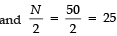∴ l = 40, f = 12, CF = 15 and h = 20

Since,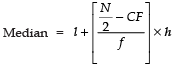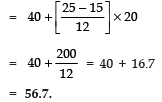Offer running on EduRev: Apply code STAYHOME200 to get INR 200 off on our premium plan EduRev Infinity!

,

,

,

,

,

,

,

,

,

,

,

,

,

,

,

,

,

,

,

,

,

;